Note: The other languages of the website are Google-translated. Back to English
ログインor今すぐ登録or

## ExcelでAND、OR、NOTを使用してIF関数を使用するにはどうすればよいですか？

Excelでは、IF関数は、セルが条件を満たすかどうかを確認し、評価結果（TrueとFalse）に基づいて異なる値を返すのに非常に役立ちます。 また、IF関数とAND、OR、NOT関数を組み合わせると、セルをチェックする条件が変わります。 ここでは、IF関数とAND関数、IF関数とOR関数の使用方法、およびExcelで複数のIF関数を組み合わせる方法を紹介します。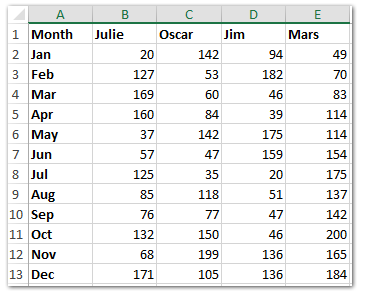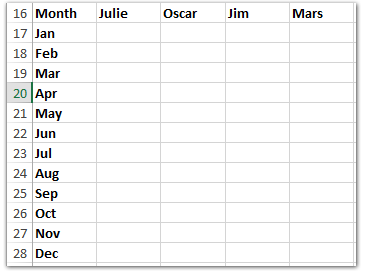#### ExcelでIF関数を使用する方法

ExcelのIF関数の基本的な形式は次のとおりです。 = IF（logic_test、value_if true、value_if_false）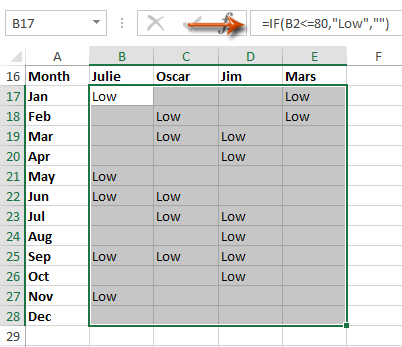#### 複数のワークシート/ワークブック/ CSVファイルをXNUMXつのワークシート/ワークブックに簡単に組み合わせる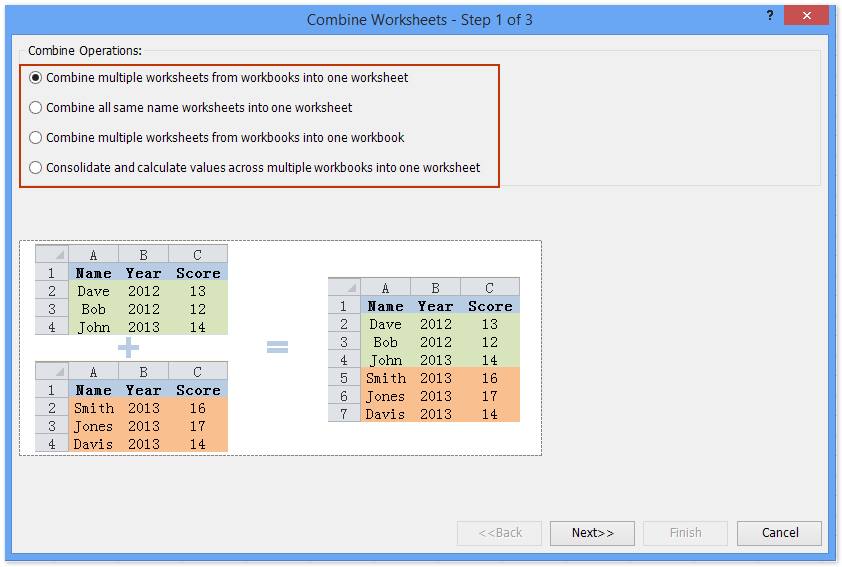#### ExcelでIf関数とAND関数を使用する方法

セルが複数の条件を満たすかどうかを同時に確認したい場合は、ExcelでIF関数とAND関数を組み合わせることができます。
ExcelのAND関数の基本的な形式は次のとおりです。 = AND（論理1、論理2、論理3、…など）

ここで、この場合、販売量が80を超え160未満であることを同時に確認し、yesの場合は「Middle」のテキストを返し、noの場合は空白を返します。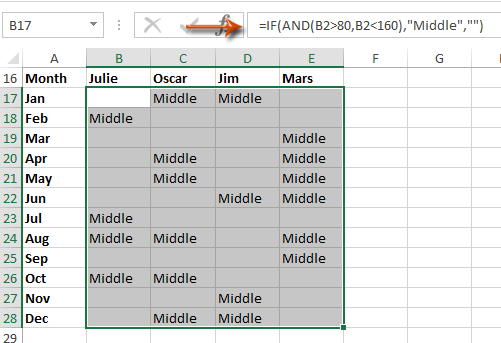#### ExcelでIF関数とOR関数を使用する方法

セルが複数の条件のいずれかを満たしているかどうかを確認する場合は、ExcelでIF関数とOR関数を組み合わせることができます。

ExcelのOR関数の基本的な形式は次のとおりです。 = OR（論理1、論理2、論理3、…など）

この例では、180を超えるまたは20未満の異常な販売量を調べます。販売量が異常な場合は、空白がない場合は「異常」のテキストを返します。#### Excelで複数のIF関数をXNUMXつの数式に組み合わせる方法

この例では、XNUMXつの式ですべての販売量を評価する場合は、複雑なIF関数を適用できます。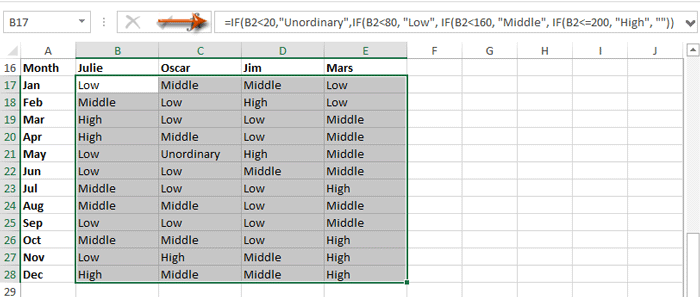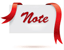数式は複雑すぎて覚えられませんか？ 数式を定型句として保存して、後でワンクリックで再利用できるようにします。 続きを読む...     無料体験

### 最高のオフィス生産性ツール

#### Kutools for Excelはほとんどの問題を解決し、生産性を80％向上させます

• 再利用: すばやく挿入 複雑な数式、チャート および以前に使用したものすべて。 セルを暗号化する パスワード付き。 メーリングリストを作成する そしてメールを送る...
• スーパーフォーミュラバー （複数行のテキストと数式を簡単に編集できます）; 読書レイアウト （多数のセルを簡単に読み取って編集する）; フィルター範囲に貼り付け...
• セル/行/列をマージする データを失うことなく; 分割セルコンテンツ; 重複する行/列を組み合わせる...重複セルを防止します。 範囲を比較する...
• [複製]または[一意]を選択します 行; 空白行を選択 （すべてのセルは空です）; スーパーファインドとファジーファインド 多くのワークブックで; ランダム選択...
• 正確なコピー 数式参照を変更せずに複数のセル。 参照の自動作成 複数のシートに; 箇条書きを挿入、チェックボックスなど...
• テキストを抽出、テキストの追加、位置による削除、 スペースを削除する; ページング小計の作成と印刷。 セルの内容とコメントを変換する...
• スーパーフィルター （フィルタースキームを保存して他のシートに適用します）; 高度な並べ替え 月/週/日、頻度など。 特殊フィルター 太字、斜体...
• ワークブックとワークシートを組み合わせる; キー列に基づいてテーブルをマージします。 データを複数のシートに分割; xls、xlsx、PDFをバッチ変換...
• 300以上の強力な機能。 Office / Excel2007-2019および365をサポートします。すべての言語をサポートします。 企業や組織に簡単に導入できます。 全機能30日間の無料トライアル。 60日間の返金保証。#### Officeタブは、タブ付きのインターフェイスをOfficeにもたらし、作​​業をはるかに簡単にします

• Word、Excel、PowerPointでタブ付きの編集と読み取りを有効にする、パブリッシャー、アクセス、Visioおよびプロジェクト。
• 新しいウィンドウではなく、同じウィンドウの新しいタブで複数のドキュメントを開いて作成します。
• 生産性が50％向上し、毎日何百ものマウスクリックが減ります。Say something here...
symbols left.
###### or post as a guest, but your post won't be published automatically.
• To post as a guest, your comment is unpublished.
· 6 months ago
I need help to make a formula
Quantity Count 1 Count 2 Count 3
10837 10874 10874 0
If count =0, make count 2-Quantity, if is <> 0, make count 3-Quantity, and if count2 and count 3 =0, make count 1-Quantity

• To post as a guest, your comment is unpublished.
· 1 years ago
I need help combining these IF formula:
IF D7<>C\$1 "OOP", "Current"
IF D7=C\$1 and S7=0, T7>0, "No Rev"
IF D7=C\$1 and S7<0, T7> 0, "Loss"
IF D7=C\$1 and S7<0, R=0, "No Cost"
IF D7=C\$1 and X7<=30%, "High GP"
IF D7-C\$1 and X7>=5%, "Low GP"
• To post as a guest, your comment is unpublished.
· 1 years ago

=IF fr2=31 (FT4>30,18000,FR4*FQ4) and IF fr2=30 (FT4>29,18000,FR4*FQ4)

i want to combine this.

• To post as a guest, your comment is unpublished.
· 1 years ago
Hi there, I need this to return the value in the column B6:B28, when either an H or an S are in the boxes I6:I28, works fine without the OR and just H, but not with the OR function, can you possibly help?=IF(AF8=I5,TEXTJOIN(",",FALSE,IF(OR(I6:I28="H",I6:I28="S"),B6:B28,"")))

Many thanks

David
• To post as a guest, your comment is unpublished.
· 1 years ago

If A1 begins with 2 zeros (00) return the number 1, if not return the number 2
• To post as a guest, your comment is unpublished.
· 2 years ago
8. To be a Bunk Leader, a staff member must have over 4 service years and have completed leadership training.
In cell L2, enter a formula using the IF and AND functions, as well as structured references to determine if Adam Moriarty can be a bunk leader.
a. The logical test in the IF function should determine if the staff member’s Service Years is greater than 4 AND the staff member’s Leadership Training status is “Yes”. Remember to use a structured reference to the Service Years and the Leadership Training columns.
b. The function should return the text Yes if a staff member meets both of those criteria.
c. The function should return the text No if a staff member meets none or only one of those criteria.
• To post as a guest, your comment is unpublished.
· 2 years ago
Hi Alice,
Supposing the number of service year is placed in Cell B2, the leadership training stats is placed in Cell C2, you can use this formula =IF(AND(B2>4,C2="Yes"),"Yes","No")
• To post as a guest, your comment is unpublished.
· 2 years ago
hello!
I need your brilliant minds to resolve a problem:
if a1=blank & b1=blank & c1=blank & d1=blank then result1
if a1=blank & b1=blank & c1=blank & d1>0 then result2
if a1=blank & b1=blank & c1>0 & d1>0 then result3
if a1=blank & b1>0 & c1>0 & d1>0 then result4
if a1>0 & b1>0 & c1>0 & d1>0 then result5
i've tried with if/and, if/or but it seams i can't get a result.

• To post as a guest, your comment is unpublished.
· 2 years ago
=IF(AND(ISBLANK(A1);ISBLANK(B1);ISBLANK(C1);ISBLANK(D1));1;IF(AND(ISBLANK(A1);ISBLANK(B1);ISBLANK(C1);D1>0);2;IF(AND(ISBLANK(A1);ISBLANK(B1);C1>0;D1>0);3;IF(AND(ISBLANK(A1);B1>0;C1>0;D1>0);4;5))))
• To post as a guest, your comment is unpublished.
· 2 years ago
Need some help with logic. I wanted to check the first cell for data if it has data then it will return that cell; if it doesn't have data then it will return a different cell. But if the second cell is blank then it should return a msg. Can you please help.
• To post as a guest, your comment is unpublished.
· 2 years ago
Hi Ahmed,
Supposing the first data in Cell A1, and the second data in Cell A2, you can apply the formula as =IF(A1="",IF(A2="","NO DATA", A2),A1)
• To post as a guest, your comment is unpublished.
· 2 years ago
IF(D15>30,"Organic Soils",IF(AND(D15>25,D15<29.99),"Clayey Soil (fine)",IF(AND(D15>20,D15<24.99),"Loamy Soil (moderately fine)",IF(AND(D15>10,D15<19.99),"Loamy Soil (medium)",IF(AND(D15>5,D15<9.99),"Loamy Soil (moderately coarse)",IF(D15<4.99,"Sandy Soil (coarse)")))))) Can you find the error?
• To post as a guest, your comment is unpublished.
· 2 years ago
A,b,c,d,e,f,g,a,y
• To post as a guest, your comment is unpublished.
· 2 years ago
Name Amount Profit Range
A 10,000
B 50,000
C 42,500
D 75,000
E 85,000
F 23,500
G 16,000
I 57,000
J 81,000

Profit Range Name
Less than 10,000 A
10,000-20,000 B
20,000-30,000 C
30,0000-50,000 D
50,0000-75,000 E
75,000-1,00,000 F

Write profit range in 1st table.
any Please say how to write formula for this
• To post as a guest, your comment is unpublished.
· 2 years ago
i required how to steel calculation as per dia (8,10,12,16,20,25,32) and cutting length no of bars and numbers etc

wise in excel format
• To post as a guest, your comment is unpublished.
· 3 years ago
how to use if condition for condition like if value of x lying in 60-80
• To post as a guest, your comment is unpublished.
· 3 years ago
Hello all,

I am trying to build an IF statement to help with our Service Level calculations. We need one Queue Name to have a different time requirement than all of the other queues. I am trying to figure out how to get the formula to count all Queue Names (Column B) to show they are within our Service Level Agreement (SLA) if they are in the queue for less than 30 seconds. However, for the Queue named TS_DX_CP_ES*, I need it to show it is within SLA if less than 90 seconds.

I currently have the following formula in place and it works for the 90 second rule but if I try to next the IF formula and change the Queue Name and Time Requirement, I get an error.

Formula that works currently =IF(AND([@[Queue Name]]="TS_DX_CP_ES*",[@[Queue time]]<0.00104166666666667),1,0)

Formula that gives a #VALUE! Error - =IF(AND([@[Queue Name]]="TS_DX_CP_ES*",[@[Queue time]]<0.00104166666666667),1,0),IF(AND([@[Queue Name]]="TS_PLU_US*",[@[Queue time]]<0.000358796296296296),1,0)
• To post as a guest, your comment is unpublished.
· 3 years ago
Hello all,

I am trying to build an IF statement to help with our Service Level calculations. We need one Queue Name to have a different time requirement than all of the other queues. I am trying to figure out how to get the formula to count all Queue Names (Column B) to show they are within our Service Level Agreement (SLA) if they are in the queue for less than 30 seconds. However, for the Queue named TS_DX_CP_ES*, I need it to show it is within SLA if less than 90 seconds.

I currently have the following formula in place and it works for the 90 second rule but if I try to next the IF formula and change the Queue Name and Time Requirement, I get an error.

Formula that works currently =IF(AND([@[Queue Name]]="TS_DX_CP_ES*",[@[Queue time]]<0.00104166666666667),1,0)

Formula that gives a #VALUE! Error - =IF(AND([@[Queue Name]]="TS_DX_CP_ES*",[@[Queue time]]<0.00104166666666667),1,0),IF(AND([@[Queue Name]]="TS_PLU_US*",[@[Queue time]]<0.000358796296296296),1,0)

• To post as a guest, your comment is unpublished.
· 3 years ago
Hi,

In a row, 3 columns with different value must show a different interpretation in the target cell.

=IF(A3>0,"Welcome",IF(B3>0,"Win",IF(C3>0,"Will","HI")))

Thanks Pinaki
• To post as a guest, your comment is unpublished.
· 3 years ago
Hi Pinaki,
In your formula, If A3 > 0, both B3 and C3 also might be greater than 0. Similarly, when B3 > 0, both A3 and C3 also might be greater than 0.

Normally, in the If function, you can set =IF(A3>0, "Welcome",””), which means when A3>0, returns “welcome”, while when A3 is not less than 0, returns nothing. In this function, the two condition A3>0 and A3<=0 are exclusive.

In my example =IF(B2<=20,"Unordinary",IF(B2<=80, "Low", IF(B2<=160, "Middle", IF(B2<=200, "High", "")))), The third condition B3<=160 includes the second condition B2<=80, and the second contains the first too.
• To post as a guest, your comment is unpublished.
· 3 years ago
Column A has Numerical Values. Column C & D have text.

If 2 or more separate Numerical Values in any row of Column A match then evaluate those Rows in Column C & D for any Text that doesn't match a given Text and count as 1. If Text does match then a value of 0 is assigned.

So if..

A1:A2 = 1

A3:A4 = 2

C1=Dog
C2=Cat
C3=Dog
C4: Horse

D1=Cat
D2=Dog
D3=Horse
D4=Dog

Since A1 & A2 match each other, evaluate C1,C2,D1,D2 and if any cells do not match either "Dog" or "Cat" assign a value of 1. In this example, since all cells contain either "Dog" or "Cat" a value of 0 would be assigned.

Furthermore,

Since A3 & A4 match each other, evaluate C3,C4,D3,D4 and if any cells do not match either "Dog" or "Cat" assign a value of 1. In this example, since cells C4 & D3 contain something other than "Dog" or "Cat" a value of 1 would be assigned.

• To post as a guest, your comment is unpublished.
· 3 years ago
Hi Cab,
To know more about the IF function, I guess this webpage is helpful.
https://support.office.com/en-us/article/IF-function-69aed7c9-4e8a-4755-a9bc-aa8bbff73be2
• To post as a guest, your comment is unpublished.
· 3 years ago
Hello,
What would the equation for the following be?

In cell K2 enter a formula using the IF and OR functions, as well as structured references, to determine if Adam Moriarty can be a group leader.
a. The IF function should first determine if the staff member’s Service Years is greater than 3 OR if the staff member’s college graduate status is “Yes”. Remember to use a structured reference to the Service Years and the College Graduate columns.
b. The function should return the text Yes if a staff member meets one or both of those criteria.
c. The function should return the text No if a staff member meets neither of those criteria.
• To post as a guest, your comment is unpublished.
· 3 years ago
Hi jessjess,
Thank you for your comment. I am sorry I can’t figure out a proper formula to meeting your conditions. To know more about the IF function, I guess this webpage is helpful.
https://support.office.com/en-us/article/IF-function-69aed7c9-4e8a-4755-a9bc-aa8bbff73be2
• To post as a guest, your comment is unpublished.
· 3 years ago
i wanna know one thing that i make a table of username and password in excel and there is a third part of them is status i wanna know that if i use a formula in a third column of status using multiple "if " and "or" for the column username where i can use both of them username or phone no then i entered password and it gives in a column of status login if username or phoneno one of them is used with password if someone enter symbols in username status failed. if someone can answer me this is for email validation in excel thanks if answered
• To post as a guest, your comment is unpublished.
· 3 years ago
Hi Haris,
• To post as a guest, your comment is unpublished.
· 3 years ago
III
II
II
II

II
II
II
II

How can I make a formula in excel -If any one value of the column is III the result shall be displayed as III, if any value of the column is II then the result shall be displayed as II if II and III not present it shall be displayed as I
• To post as a guest, your comment is unpublished.
· 3 years ago
How would I write this into one function?

If D26 and E26 are blank, then C26-L7
If D26 is not blank and E26 is, then D26-L7
If E26 is not blank, then E26-L7
• To post as a guest, your comment is unpublished.
· 3 years ago
=IF(AND(D26="",E26=""),(C26-L7),IF(AND(D26<>"",E26=""),D26-L7,E26-L7))
• To post as a guest, your comment is unpublished.
· 3 years ago
I am trying to write a formula to look at

1. Is the figure either over £1000, or under -£1000

2. Plus is the next cell over 55% or more

so far I have =OR(M4>=1000,M4<=-1000) which pulls in the over or under £1000 plus or minus but am unsure how to add the second criteria presume its an AND(N2>=55%) but when I add it to the formula it doesnt work.
• To post as a guest, your comment is unpublished.
· 3 years ago
Hi Michelle,
• To post as a guest, your comment is unpublished.
· 3 years ago
Hi. How can I write this as formula?

If year is equal to current year, add 1.
if year is previous year, add 1 OR start 1.
if year is 2 years prior, add 1 OR start 1.
• To post as a guest, your comment is unpublished.
· 3 years ago
Hi Everyone, I need to write a formula for the following:

IF A1 = "BOTTOMS" AND A9 <14, then 14, otherwise A9

OR A1 = "TOPS" AND A9 <5, then 5, otherwise A9
• To post as a guest, your comment is unpublished.
· 3 years ago
Hi FG，
You can’t create a formula to meeting one of both your conditions in Excel.
• To post as a guest, your comment is unpublished.
· 3 years ago
Hi Kelly!

I actually figured out a workaround!

=ROUND((IFERROR((IF(OR(AND(\$I9="BOTTOMS",BL9>=\$BY\$3),AND(\$I9="TOPS",BL9>=\$BY\$4)),BL9,VLOOKUP(\$I9,\$BX\$3:\$BY\$4,2,FALSE))),0)),0)

I9 - populates "BOTTOMS" or "TOPS" dependant on merchandise code entered
BL9 - minimum set defined by store indice
BY9 - minimum set defined by buyer (i.e the 14 units for bottom and and the 5 units for tops)
BX\$3:\$BY\$4 - vlookup to minimum set defined by buyer if if statement is false
• To post as a guest, your comment is unpublished.
· 3 years ago
Hello! I am currently creating a template that will provide me a value depending on the score of the trainee.

I was hoping I can add a value of 5 for any team member who gets a certain quiz %

Here's what I'm thinking.

5= 100%
4= 99.9% to 90%
3= 90% to 85%
2= 85% to 80%
1= <79.99

I hope you guys can help me with the formula
• To post as a guest, your comment is unpublished.
· 3 years ago
Hello, I have a pretty complex formula I am trying to write and it is killing me to admit defeat on this one. So far I have:

=IF(AND(B2="Prudential",OR(C2={"10 Year Term","15 Year Term","20 Year Term","30 Year Term"})),D2*0.75,(IF(C2="UL",D2*0.8,"0")))

and it works just fine. However, I need to add about 37 more companies with their own commission structures to it. I am pretty sure I just need one monster OR statement with a bunch of nested IFs thrown in I just am at my wits end on figuring out exactly where to place them. Any help would be amazing. Thank you!
• To post as a guest, your comment is unpublished.
· 3 years ago
Hi Dan,
Your question is still unclear. you might to explain more by providing a solution for at least 2 companies. However, just help to shorten your OR function a bit. OR(C2={"10","15","20","30"}&" Year Term").
• To post as a guest, your comment is unpublished.
· 3 years ago
=IF(OR((I2=(T2+U2),(I2=(U2+V2)),"Closed","OPEN")
HOW TO MAKE THIS RIGHT?
• To post as a guest, your comment is unpublished.
· 3 years ago
=IF(OR(I2=T2+U2,I2=U2+V2),"CLOSED","OPEN")
• To post as a guest, your comment is unpublished.
· 3 years ago
Im trying to do IF(OR(A1=1;AND(A2=1;A3=1)))
• To post as a guest, your comment is unpublished.
· 3 years ago
Hi Mirko,
Thank you for your comment. What calculation result do you want to get? Could you tell me more about your problem?
• To post as a guest, your comment is unpublished.
· 3 years ago
I'm trying to make the formula return 4 possible answer which is (R-regular,A-absent,L-late,OT-overtime). My basis for regular is if the value of the cell is "8". So this is the formula that I made so far =IF(H16>7.9,"OT",IF(H16<7.9,"L",IF(H16=8,"R","A"))). It only returns (OT and L) something is very wrong and i can't figure it out. I'm just new to excel so please help me
• To post as a guest, your comment is unpublished.
· 3 years ago
Hi Dan,
Try this formula =IF(H16=8,"R",IF(H16<7.9,"OT","L"))
• To post as a guest, your comment is unpublished.
· 3 years ago
I'm trying to make the formula return 4 possible answer which is (R-regular,A-absent,L-late,OT-overtime). My basis for regular is if the value of the cell is "8". So this is the formula that I made so far =IF(H16>7.9,"OT",IF(H16<7.9,"L",IF(H16=8,"R","A"))). It only returns (OT and L) something is very wrong and i can't figure it out. I'm just new to excel so please help me.
• To post as a guest, your comment is unpublished.
· 3 years ago
Try this: =IF(OR(H16=0,H16=""),"A",IF(H16=8,"R",IF(H16<7.9,"L","OT")))
• To post as a guest, your comment is unpublished.
· 3 years ago
how to determine the age of the cell ? is this formula right =IF(I2<30,"future payment",AND(IF(I2>30<=45,"30-45days",AND(IF(I2>45<=60,"45-60days",AND(IF(I2>60,"61+days
",0)))))))
• To post as a guest, your comment is unpublished.
· 3 years ago
Hi pramod,
You cannot use I2>30<=45 in formula directly. I have changed the formula for you: =IF(I2<=30,"FUTURE PAYMENT",IF(B2<=45, "30-45 DAYS", IF(B2<=45, "45-60DAYS", IF(B2<=60, "60+DAYS", ""))))
• To post as a guest, your comment is unpublished.
· 3 years ago
I have charges calculation for eg. charges amount is 0 then next column should be 0. if charges is more than 1 and less than 250 then next column should be 250. If charges is more than 250 then actual charges should come in next column(252)
• To post as a guest, your comment is unpublished.
· 4 years ago
how do i make this formulas talk to one another:

=IF(OR(AND(MID(K2,6,1)="N",(MID(K2,6,1)="C"),(MID(K2,6,1)="H"),(MID(K2,6,1)="I"),(MID(K2,6,1)="B"),(MID(K2,6,1)="F"),(MID(K2,6,1)="L"),(MID(K2,6,1)="M"),(MID(K2,6,1)="P"),(MID(K2,6,1)="R"),(MID(K2,6,1)="P"),ISTEXT(G2)="61"),OR(AND(RIGHT(K2,2)=G2))),"Good","Review")

=IF(AND(RIGHT(K2,2)=G2),"Good","Review")

or =IF(NOT(OR(AND(MID(K2,6,1)="N",(MID(K2,6,1)="C"),(MID(K2,6,1)="H"),(MID(K2,6,1)="I"),(MID(K2,6,1)="B"),(MID(K2,6,1)="F"),(MID(K2,6,1)="L"),(MID(K2,6,1)="M"),(MID(K2,6,1)="P"),(MID(K2,6,1)="R"),(MID(K2,6,1)="P"),ISTEXT(G2)="61"),(RIGHT(K2,2)=G2))),"Good","Review")